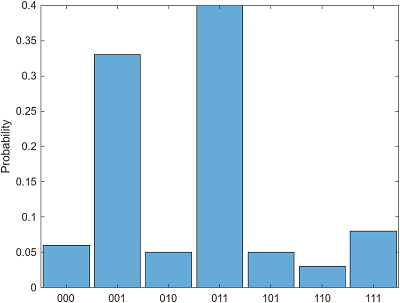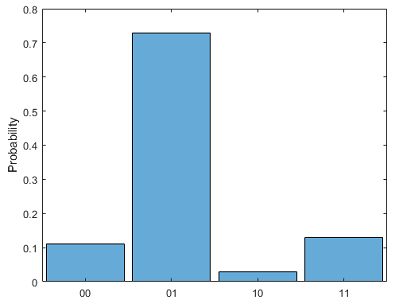# quantum.gate.QuantumMeasurement class

Package: quantum.gate

Measurement result of quantum circuit

Since R2023a

Installation Required: This functionality requires MATLAB Support Package for Quantum Computing.

## Description

A `QuantumMeasurement` object represents the measurement result of a quantum circuit, either by running the circuit remotely on a quantum device or by simulating the circuit locally with random sampling. This object contains information about the counts of all measured states of the n qubits of the circuit.

## Creation

• Use `run` to run a circuit remotely on a quantum device and fetch the finished task using `fetchOutput` to return a `QuantumMeasurement` object.

• Use `randsample` on a `QuantumState` object that represents the quantum state of the qubits of a circuit. `randsample` randomly samples this state locally (with a specified number of shots) and returns the measurement result as a `QuantumMeasurement` object.

## Properties

expand all

Measured states in the Z basis, returned as a string array.

#### Attributes:

 `GetAccess` `public` `SetAccess` `private`

Counts of measured states, returned as a vector of positive integers. Each element of this vector represents how often each state was measured.

#### Attributes:

 `GetAccess` `public` `SetAccess` `private`

Number of qubits, returned as a positive integer scalar.

#### Attributes:

 `GetAccess` `public` `SetAccess` `private`

expand all

## Examples

collapse all

Create a quantum circuit that consists of three x-axis rotation gates. The first gate acts on qubit 1 with rotation angle `pi/4`, the second gate acts on qubit 2 with rotation angle `pi/2`, and the third gate acts on qubit 3 with rotation angle `3*pi/4`.

```g = rxGate(1:3,pi/4*(1:3)); c = quantumCircuit(g);```

Simulate this circuit using the default initial state, where all qubits are in the $|0〉$ state. After running the circuit, randomly sample the quantum state with 100 shots and return the resulting simulated measurement.

```s = simulate(c); m = randsample(s,100)```
```m = QuantumMeasurement with properties: MeasuredStates: [7×1 string] Counts: [7×1 double] NumQubits: 3```

Show the counts of the measured states.

`table(m.Counts,m.MeasuredStates,VariableNames=["Counts","States"])`
```ans = 7×2 table Counts States ______ ______ 6 "000" 33 "001" 5 "010" 40 "011" 5 "101" 3 "110" 8 "111" ```

Plot the histogram of the measurement result to show each measured state and its estimated probability.

`histogram(m)`You can also specify which qubits to plot in the histogram. The histogram shows the measured states of the specified qubits (where the other qubits can be in any state) and their corresponding probability distributions (where the probabilities of the other qubits being in any state are combined).

For example, specify qubits 1 and 3 to plot in the histogram. This histogram shows the measured states $|00〉$, $|01〉$, $|10〉$, and $|11〉$, where their corresponding probabilities are `0.11`, `0.73`, `0.03`, and `0.13`.

`histogram(m,[1 3])`Query each measured state and its estimated probability.

`[states,probabilities] = querystates(m)`
```states = 7×1 string array "000" "001" "010" "011" "101" "110" "111" probabilities = 0.0600 0.3300 0.0500 0.4000 0.0500 0.0300 0.0800```

You can also specify which qubits to query when using `querystates`.

`[states,probabilities] = querystates(m,[1 3])`
```states = 4×1 string array "00" "01" "10" "11" probabilities = 0.1100 0.7300 0.0300 0.1300```

Create a quantum circuit that consists of a Hadamard gate and a controlled X gate to entangle two qubits.

```gates = [hGate(1); cxGate(1,2)]; c = quantumCircuit(gates);```

Connect to a remote quantum device through AWS. Create a task that runs the circuit on the device.

```dev = quantum.backend.QuantumDeviceAWS("Lucy"); task = run(c,dev);```

Wait for the task to finish. Retrieve the result of running the circuit on the device.

```wait(task) m = fetchOutput(task)```
```m = QuantumMeasurement with properties: MeasuredStates: [4×1 string] Counts: [4×1 double] NumQubits: 2```

Show the measurement result of running the circuit. Due to the noise in the physical quantum device, the $|01〉$ and $|10〉$ states can appear as measurements.

`table(m.Counts,m.MeasuredStates,VariableNames=["Counts","States"])`
```ans = 4×2 table Counts States ______ ______ 46 "00" 9 "10" 3 "01" 42 "11" ```

## Version History

Introduced in R2023a